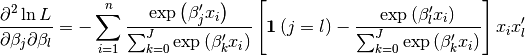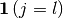# statsmodels.discrete.discrete_model.MNLogit.hessian¶

MNLogit.hessian(params)[source]

Multinomial logit Hessian matrix of the log-likelihood

Parameters: params : array-like The parameters of the model hess : ndarray, (J*K, J*K) The Hessian, second derivative of loglikelihood function with respect to the flattened parameters, evaluated at params

Noteswhereequals 1 if j = l and 0 otherwise.

The actual Hessian matrix has J**2 * K x K elements. Our Hessian is reshaped to be square (J*K, J*K) so that the solvers can use it.

This implementation does not take advantage of the symmetry of the Hessian and could probably be refactored for speed.